Li, X., Qian, W., Cheng, L., and Chang, L. (2020). "A coupling model based on grey relational analysis and stepwise discriminant analysis for wood defect area identification by stress wave," BioRes. 15(1), 1171-1186.

#### Abstract

Based on the experimental idea of reverse simulation, a quantitative area of hole was excavated at the sectional center of a wood specimen. The excavation area was 1/32S, 1/16S, 1/8S, 1/4S, and 1/2S (where S represents cross-sectional area of the complete specimen) and stress wave nondestructive testing of six sensors was performed. The stress wave propagation paths were statistically summarized to obtain the stress wave propagation velocity (Va) for two adjacent sensors, the stress wave propagation velocity (Vb) for two separated sensors, and the stress wave propagation velocity (Vc) for two opposite sensors. Furthermore, by analyzing the advantages and disadvantages of grey relation and stepwise discriminant model when both of them were used alone, a coupling model generated from them was established to dispose the test data. The attenuation ratios Ψa, Ψb, and Ψc of stress wave under three propagation paths and their relation ratios Va/Vb, Vb/Vc, and Va/Vc, a total of six groups of measured data, were selected as discriminant factors for the hole area grade of the wood specimen. The verification results showed that the discriminant accuracy of the coupling model was 100%, and it was concluded that the attenuation ratio (Ψb) of the stress wave propagation velocity for two separated sensors had the strongest discriminant ability against cross-sectional area of the specimen.

A Coupling Model Based on Grey Relational Analysis and Stepwise Discriminant Analysis for Wood Defect Area Identification by Stress Wave

Xin Li,a Wei Qian,b,* Liting Cheng,b and Lihong Chang c

Based on the experimental idea of reverse simulation, a quantitative area of hole was excavated at the sectional center of a wood specimen. The excavation area was 1/32S, 1/16S, 1/8S, 1/4S, and 1/2S (where represents cross-sectional area of the complete specimen) and stress wave nondestructive testing of six sensors was performed. The stress wave propagation paths were statistically summarized to obtain the stress wave propagation velocity (Va) for two adjacent sensors, the stress wave propagation velocity (Vb) for two separated sensors, and the stress wave propagation velocity (Vc) for two opposite sensors. Furthermore, by analyzing the advantages and disadvantages of grey relation and stepwise discriminant model when both of them were used alone, a coupling model generated from them was established to dispose the test data. The attenuation ratios ΨaΨb, and Ψc of stress wave under three propagation paths and their relation ratios Va/VbVb/Vc, and Va/Vc, a total of six groups of measured data, were selected as discriminant factors for the hole area grade of the wood specimen. The verification results showed that the discriminant accuracy of the coupling model was 100%, and it was concluded that the attenuation ratio (Ψb) of the stress wave propagation velocity for two separated sensors had the strongest discriminant ability against cross-sectional area of the specimen.

Keywords: Stress wave; Defect area identification; Grey relational analysis; Stepwise discriminant analysis; Coupling model

Contact information: a: College of Architecture and Art, North China University of Technology, Beijing 100144, China; b: Beijing Engineering Technology Research Center for Historic Building Protection, Beijing 100022, China; c: College of Urban & Rural Development, Beijing University of Agriculture, Beijing 102206, China; * Corresponding author: legend622@163.com

INTRODUCTION

Wood is an excellent building material with high specific strength, good thermal performance, easy processing, resource renewability, and other advantages. However, as a biomass material, wood has anisotropic mechanics characteristics, and it easily forms internal defects under the influence of heredity, environment, and human factors, resulting in a decrease in mechanical properties (Wodzicki 2001; Frühwald 2011; Duan et al. 2014). Therefore, it is particularly important to determine the health of wood, especially wooden building components that were in use. Nondestructive testing technology has been widely used in the field of wood detection in recent years. The basic idea of this technology is to use different physical and mechanical or chemical properties of materials to effectively test relevant properties of the target object, such as shape, displacement, defects, mechanical properties, etc., without destroying the internal and external structure and performance of the tested object (Zhu et al. 2011). Nondestructive testing technology can not only prevent artificial trauma and damage to wood, but also it can provide a quick and accurate judgment on the defects inside the wood (Halabe et al. 1997; Bernabei and Bontadi 2012; Yue et al. 2016).

The stress wave technique is one of the nondestructive testing techniques often applied in wood testing; it boasts the advantages of portability, causing no wood shape limitation and minimal surface invasion, yielding visually visible results, avoiding the need for coupling agents, etc. (Balayssac et al. 2011). The acoustic properties of wood are the physical basis of stress wave detection. The working principle is to use the propagation time detected between acceleration sensors, for the known propagation distance and the calculated stress wave propagation velocity; where there are defects in the wood, such as decay, cavity, or cracks, the velocity of the stress wave detected at both ends of the defect will decrease. Cavities in particular will have a noticeable effect on the stress wave propagation in the wood, resulting in the extension of propagation distance of the stress wave and the increase of propagation time (Deflorio et al. 2008; Xu and Wang 2014). Through matrix transformation and reconstruction of the supporting software of detection equipment, the image reconstruction of the fault plane of wood will reveal the internal defects of the wood (Liu et al. 2018; Sarnaghi and Van de Kuilen 2019).

Relevant studies have shown that the prediction accuracy of stress wave detection on wood internal defects can be effectively improved by analyzing the propagation law of stress wave in wood media, conducting data mining and deep learning on the propagation speed of stress waves, and incorporating corresponding mathematical analysis models. The adopted methods include artificial neural networks (Qi and Mu 2006), support vector machine (Wang et al. 2015), the Shapley value (Chang et al. 2016), four sub-step explicit time integration (Kwon and Lee 2017), optimized convolution neural networks (Liu et al. 2019), etc. Each of these methods has its own characteristics but also has certain limitations; for example, the artificial neural networks method has a slow convergence speed and unstable calculation results, the support vector machine method is difficult to determine reasonable weight parameters, etc. Therefore, this study attempts to examine the grey relational method and the stepwise discriminant method as the basic models, proposes a coupling judgment idea, and designs and establishes the coupled wood internal defect area grade discriminant model based on the data of propagation velocity of the stress wave in wood.

EXPERIMENTAL

Materials

The wooden column members dismantled from the ancient building renovation project in Beijing, northern China were selected and identified as Pinus tabulaeformis by tree species identification. The cross-sectional shape was approximately circular. Six specimens with a height of 100 mm were taken from the middle position as research objects of this experiment, named TP1 to TP6. The average cross-sectional area of TP1 to TP6 was = 535 cm2, and the average diameter (d) was 280 mm. The specimens were placed in an artificial climate box until a moisture content of 12%, to simulate the moisture content state of the wood member in natural environment, as shown in Fig. 1.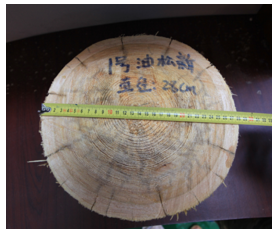Fig. 1. The experimental material (Pinus tabuliformis)

Test Design

The FAKOPP 3D Acoustic Tomograph (FAKOPP Enterprise, Agfalva, Hungary) was used in this test. This instrument is able to detect the size and location of decayed and hollow parts in the wood non-destructively, utilizing the basic measurement principle that sound velocity drops if there is a hole between two sensors. During the test, the researchers evenly arranged six stress wave detecting sensors along the perimeter of the specimens to measure the stress wave propagation velocity of each specimen under the state of no cross-sectional hole (initial working condition), and ultimately selected three paths: the velocity for two adjacent sensors (Va), including V12V23V34V45V56, and V61 and whose arithmetic mean was recorded as Va0; the velocity for two separated sensors (Vb), including V13V24V35V46V51, and V62 and whose arithmetic mean was recorded as Vb0; and the velocity for two opposite sensors (Vc), including V14V25, and V36 and whose arithmetic mean was recorded as Vc0, as shown in Fig. 2. The Va0Vb0, and Vc0 were the initial working condition values under the three propagation paths.

Further, the researchers excavated through-holes of a quantitative area at the section centers of TP1 to TP6, detected the stress waves correspondingly, and repeated the above-mentioned detection process as the excavation area gradually expanded to form five test conditions. The excavation areas were S= 1/32SS= 1/16SS= 1/8SS= 1/4S, and S= 1/2S, and the corresponding hole diameters were d= 50 mm, d= 70 mm, d= 100 mm, d= 140 mm, and d= 200 mm, respectively (due to the error of manual operations, the excavation diameters were rounded to the whole number of 10 digits). The researchers obtained the VakVbk, and Vck (= 1, 2, 3, 4, and 5) under the condition of holes of different areas and calculated their ratio Ψn with the initial comparison values Va0Vb0, and Vc0, which is their respective wave velocity attenuation ratios, as shown in Eq. 1,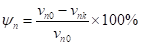(1)

where Vn(a, b, or c) is the average initial comparison value of the stress wave propagation velocities under the three paths, and Vnk (a, b, or c= 1, 2, 3, 4, or 5) is the average value of the stress wave propagation velocities of the three paths under the hole areas of 1/32S, 1/16S, 1/8S, 1/4S, and 1/2S, respectively.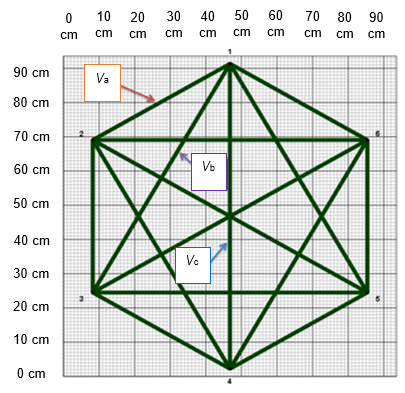Fig. 2. Three propagation paths of stress wave velocities among the timber cross-section

CALCULATION MODEL

Principle of Coupling Model

Grey relational analysis (GRA) is a multi-factor statistical analysis method based on the sample data of each factor to use the grey relation degree to describe the strength, size, and order of the relations among factors. A greater relation degree means more closer between the two factors, whereas a lower degree means more deviation between the two factors (Biswas et al. 2014). Because GRA is a quantitative analysis method based on qualitative analysis, when it was applied to the discrimination of wood defect area grade, the discriminant result was greatly affected by the division of the reference sample individual type. In addition, the model needed to determine the optimal value of each indicator, which was subjective and difficult to determine the optimal value of some indicators. Therefore, when the defect area grade of the pending sample was highly related with the individual reference sample, but was contrary to the overall relation trend, it was easy to misjudge (Yang et al. 2008).

The basic concept of stepwise discriminant analysis (SDA) is to introduce variables with the most significant discriminant ability into the discriminant function at each step, detect significance of the discriminant ability of the variables that have been selected stepwise, and remove the variables whose discriminant ability is no longer significant from the discriminant function until all the variables available for selection can be neither selected nor removed (Meng et al. 2010; Celeux et al. 2019). Compared with ordinary discriminant methods, SDA has the advantages of a small amount of needed calculation and high accuracy. However, in the process of identifying wood defect area grade, different types of training sample combinations affected the screening of variables, and although the posterior probability of multiple results was high, the liquefaction hazard grade could not be determined accurately due to inconsistent discriminant types.

In this study, the establish idea of coupling model is: the variables were preliminarily screened by GRA firstly, and then the variables that exhibited the strongest discriminate ability were chosen by SDA. Before establishing a coupling model, the following definitions were made: Unknown type pending sample sequence X0(k) = {x0(1), x0(2), x0(3),…x0(n)}, known type pending sample sequence Xi(k) = {xi(1), xi(2), xi (3),…xi (n)}, (= 1, 2, 3, …, M), where M represents the number of known types of sample.

Grey Relational Analysis Model

In Grey Relation Analysis, the main goal is to calculate the relation degree between the pending samples and the reference sample. The following steps are required:

(1) The reference sequence X0(k) and the comparison sequence X1(k) were determined based on the analysis of existing problems.

(2) Dimensionless processing of the original sequence was conducted. Because the dimensions of the indicators in the original data are not uniform, some indicators even do not have dimensions. Therefore, the original sequence should be dimensionless before calculation to obtain comparable data sequences. The dimensionless processing includes methods such as maximization, minimization, and averaging.

(3) The relation coefficient was calculated. For the reference sequence X0(k) and the comparison sequence X1(k), the following relation can be used to show the difference between the comparison curve and the reference curve at each point (moment),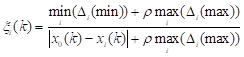(2)

where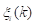represents the relative difference of the comparison curve X1 and the reference curve X0 at the kth moment, called the relation coefficient of Xi to Xat the kth moment, where ρ represents the resolution ratio, generally between 0 and 1, and a smaller ρ value results in greater resolution,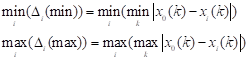(3)

where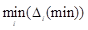and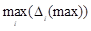represent the minimum and maximum differences of two levels at different moments, respectively.

1. The relation degree was calculated. Considering that there were many calculation results of relation coefficient and that the information was too scattered to facilitate comparison, it was necessary to concentrate the relation coefficients at each moment into one value, that is, the relation degree. The general expression of relation degree is: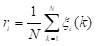(4)

A bigger ri value results in closer development trends of the reference sequence X0(k) and the comparison sequence Xi(k).

1. The relation degree was sorted. The relation degree between the reference sequence X0(k) and the comparison sequence Xi(k) was then sorted to obtain a relation sequence.

Discriminant Type Screening

The relation degree of X0(k) with each sequence in Xi(k) was obtained by calculation, and the grouping type of each sample in Xi(k) was known; therefore, the type relation sorting sequence Gof X0(k) was obtained according to relation sequence. The dG is defined as the number of input types to go to the next stepwise discriminant analysis, and dG ≥ 2. The method of determining dG was as follows: First calculate the arithmetic mean of the relation degree between X0(k) and Xi(k), with Eq. 5 as follows: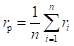(5)

Introduce the type greater than rp in the relation sorting sequence Gto the SDA, obtain quantity value of the type dG, and remove the remaining types. When d< 2 was calculated from this, it should take dG = 2.

The type sequence Gp after screening could be obtained by the above method. According to the sequence, screen the samples corresponding to relevant types in Xi(k) to form a new sequence Xj(k), where j = (1, 2, 3,…,N), and introduce X0(k) and Xi(k) to the stepwise discriminant analysis, thus determining the discriminant type of X0(k).

Stepwise Discriminant Analysis

After type screening, the number of types corresponding to the type sequence Gp was G‘, and then it was necessary to perform G‘ group discrimination against X0. Each group has a known type of sample of n’gg = 1, 2, …,G’）, so the total number of known type of samples was n’ = n’1+n’2+…+n’g. The samples were stepwise discriminated through the following steps:

(1) Let the raw data after category screening be xigk, and then the average value of the data of each groupxig, the general averagexi, the intra-group dispersion matrix W, and the general dispersion matrix are calculated.

(2) Stepwise select or remove variables. Let the l-step calculation be performed and g variables xi1xi2, …, xig selected, adopt the known W(l) and T(l), and the discriminant ability of all variables at Step + 1 are calculated first, including the unselected variables and the selected variables, with the formula as follows:

Unselected variables: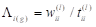, ≠ i1, i2, …, ig (6)

Selected variables: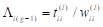, i1, i2, …, ig (7)

Secondly, whether the selected variables should be removed was judged, where the corresponding xr represents the lowest discriminant ability. The F test about removing xshould be performed, with the formula as follows: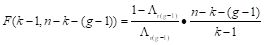(8)

If the discriminant ability of xr is insignificant, the variable should be removed, otherwise conduct the next step of calculation. In addition, examine the newly selected variables, select the variable xr with the strongest discriminant ability and conduct tests, with the formula as follows: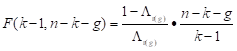(9)

If the discriminant ability of xr is significant, transform W(l) and T(l), otherwise the above steps are repeated. When no new variables could be introduced and no variables could be removed, the process of stepwise discrimination ends; and at this time, the discriminant coefficient could be calculated with W(l) and the discriminant formula could be obtained accordingly.

(3) The discriminant coefficient is calculated. If the variable screening ends at Step l, and the selected variables are xi1xi2, …, xig, then the calculation should be performed as follows:

① According to the calculation of discriminant coefficient with W(l), the formulas are as follows,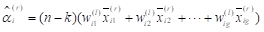i1, i2, …, ig (10)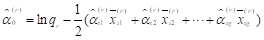(11)

where = 1, 2, …, k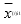is the average value of selected variable xig in Category r, and qr was the prior probability.

② Discriminant effect tests on the selected variables are performed,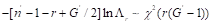was adopted to test the discriminant effect of the general G‘, and conduct significance test against selected variables according to the F approximation formula corresponding to Λr. The discriminant calculation of any two groups of l and f is calculated as follows,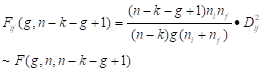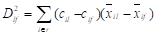(12)

where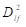is the Mahalanobis distance between the group of and f, and if Flf > Fα, it means that the discriminant effect of the two groups of l and f is good.

(4) Discriminant classification. If the discriminating effect of the variables is significant, any individual x(x1x2, …, xr) in the pending sample can be conducted discriminant classification stepwise with the following discriminant function: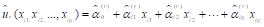(13)

If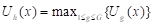, then classify x to Group h, and then calculate the posterior probability P(h/x), with the formula as follows: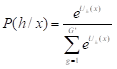(14)

RESULT AND DISCUSSION

Test Data Analysis

The ArborSonic 3D 5 for Windows (FAKOPP Enterprise, 5.3.121, Agfalva, Hungary), which was a bundled software with the stress wave detection equipment, could perform matrix transformation and graphic processing on the collected stress wave propagation velocity data to obtain computer simulation images of internal conditions of the sections of wood members displayed in different colors. The comparison of specimen state and stress wave detection image under five kinds of hole area conditions is as shown in Fig. 3. According to the image, the stress wave detection image could reflect the true state of the specimen more intuitively and accurately.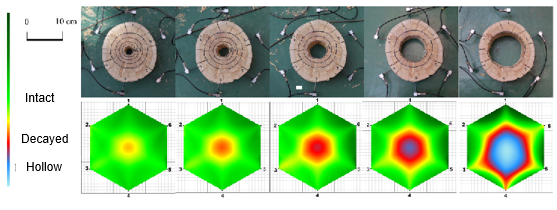Fig. 3. Comparison of actual hole area and imaged area by stress waves

Further, according to the test flow design, values of the stress wave propagation velocities under six test conditions are as shown in Table 1. The analysis showed that: (i) In the initial working condition (no hole), the data distribution of Va, Vb, and Vc was unnoticeably different, and the overall coefficient of variation was only 3.48%. (ii) With the expansion of the excavated hole area, the propagation velocities of the three paths were attenuated, but the degree of attenuation was different; the attenuation of Vc was the fastest, followed by Vb, and then Va. The overall coefficient of variation showed an increasing trend, reaching a maximum of 26.28%. (iii) When the cross-sectional hole area was less than 1/4S, the attenuation trend of Va was not obvious, and the maximum attenuation coefficient was only 3.19%; when the cross-sectional hole area was 1/2S, the attenuation trend of Va was noticeable, and the attenuation coefficient reached 15.70%; when the cross-sectional hole area was less than 1/16S, the attenuation trend of Vb was not obvious, and the maximum attenuation coefficient was only 3.15%; when the cross-sectional hole area was larger than 1/8S, the attenuation trend of Vb became more noticeable, and the maximum attenuation coefficient reached 31.40%. (iv) The cross-section hole always had an effect on Vc. With the expansion of the cross-sectional hole area, the attenuation of Vshowed a linear trend. When the cross-sectional hole area was 1/32S, the attenuation coefficient of Vc had reached 11.02% and maximally reached 55.59%.

Table 1. Data Analysis of Stress Wave Velocity Propagation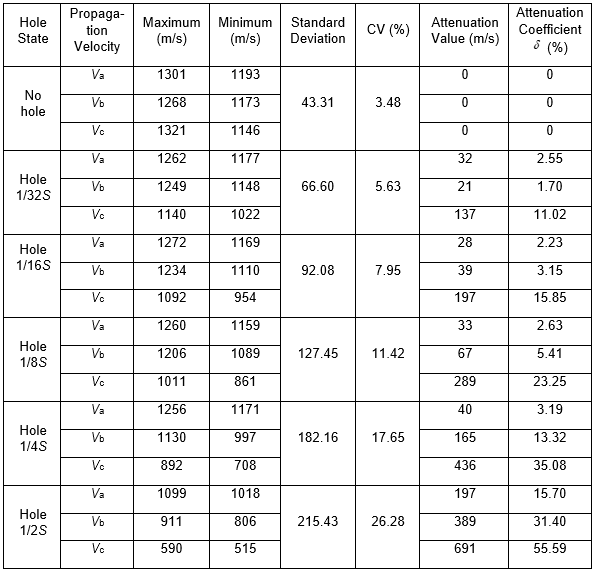Data Preparation

Further, the grey relation-stepwise analysis coupling model was introduced to optimize the influence law of the stress wave propagation velocity on the cross-sectional hole area and the discriminant accuracy. Firstly, in this study, based on analysis of the attenuation law of the measured stress wave propagation velocities under the three paths, six parameters were selected as discriminant factors from two aspects, including the attenuation ratios of stress wave rates under three propagation paths ΨaΨb, and Ψc and the mutual ratio of stress wave rates under three propagation paths Va/VbVb/Vc, and Va/Vc. Meanwhile, the cross-sectional hole area grades were defined as no-hole (I), 1/32S (II), 1/16S (III), 1/8S (IV), 1/4S (V), and 1/2S (VI).

A total of 36 groups of data of Specimens TP1 to TP6 were measured as sample data under six test conditions, where the six working conditions of TP1 served as the pending samples, recorded as G1 to G6, and the remaining 30 groups of data were taken as the reference samples of discriminant models, with the data as shown in Table 2.

Table 2. Data Preparation for Model Building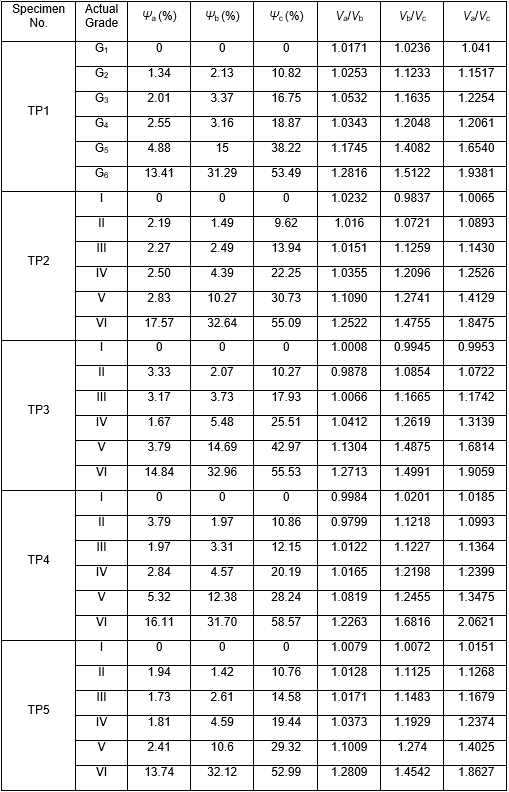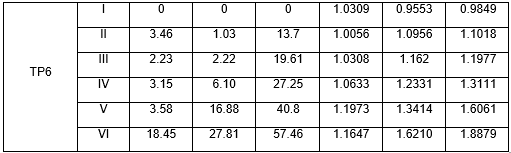Model Construction and Calculation

Based on the GRA model described above, the relation degree between the pending sample data G1 to G6 and the reference sample data was calculated to obtain relation degree ranking of the hole area grade, as shown in Table 3. In this study, the resolution ratio ρ was taken as 0.5.

After calculation of the relation degree between the pending sample and the reference sample was completed, the discriminant type was screened. In this study, the arithmetic mean rof the relation degree was selected as the threshold for the relation degree of type screening. Taking Pending Sample G1 as an example, the arithmetic mean of the relation degree in Table 3 was r= 0.7862. Therefore, in Sequence G1, the discriminant result with a relation degree value greater than 0.7862 was retained and introduced to the next stepwise discriminant model, and the remaining was discarded. The screening results of the discriminant types of G1 to G6 as obtained with this method are shown in Table 4.

Table 3. Grey Relational Analysis Results of Samples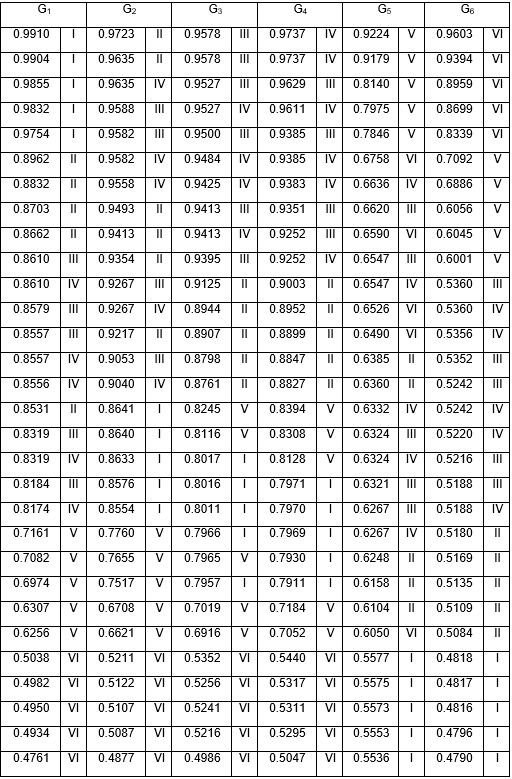Table 4. Group Screening Results of Discriminant Analysis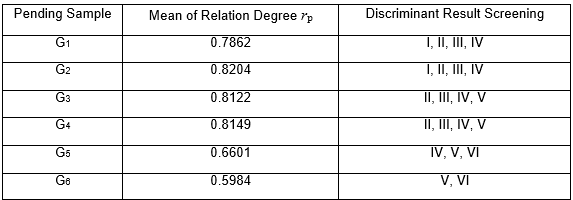After the discriminant grades of samples were screened, the hole area grades with a strong correlation with the samples were determined. Through introducing the stepwise discriminant model, the six discriminant parameters were further screened to select the parameters with the most noticeable discriminant ability. With G3 as an example and ΨaΨbΨcVa/VbVb/Vc, and Va/Vc as input variables, the discriminant grades II, III, IV, and V obtained after the previous screening were used as output variables to establish a stepwise discriminant model and gave a noticeable level = 0.05. After the stepwise discriminant calculation, the variables Ψb and Ψc with the most noticeable discriminant ability were obtained as the selected variables, and the discriminant function was as follows:

II = ln ½ + 0.012 u2 + 0.107 u3 – 1.386 = -0.246

III = ln ½ – 0.924 u2 + 0.171 u3 – 9.311 = 6.496

IV = ln ½ + 0.719 u2 + 1.959 u3 – 27.443 = 4.793

V = ln ½ + 3.305 u2 + 1.240 u3 – 44.248 = -13.033

It can be seen from the calculation results that III was the largest, so it was determined that the discriminant grade of Pending Sample G3 was Grade III and the posterior probability was 0.8451, which were also consistent with those of the actual hole area grade. Similarly, the hole area grade discrimination of the remaining pending samples was completed, and the results shown in Table 5.

Table 5. Results of Stepwise Discriminant Analysis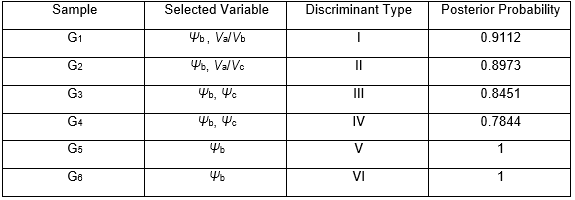Discriminant Result Analysis

When the grey relation discriminant model was used alone, it could be seen from the ranking of the relation degree values that the discriminant grades of Samples G1, G5, and G6 were consistent with the actual grades, only because the ranking grades of the Top 5 ranks were the same as the actual ranking. Pending Samples G2, G3, and G4 had a certain degree of uncertainly in discriminant, because only the ranking grades of the Top 2 ranks were the same as the actual ranking. In general, when the grey relation discriminant model was used alone, the actual grade of the cross-sectional hole area of the wood could be judged with the six discriminant parameters ΨaΨbΨcVa/VbVb/Vc, and Va/Vc, with the most unlikely two grades screened out. When there was no hole and the hole area was ≥1/4S, the discriminant accuracy of the model was high; and when the hole area was < 1/4S, the discriminant accuracy was general.

After introducing the stepwise discriminant model analysis, it could be seen that when the cross-sectional hole area of the wood was ≤ 1/32S, the discriminant ability of Ψb and Va/Vc were the most noticeable; when the cross-sectional hole area was ≥ 1/16S but ≤ 1/8S, the discriminant ability of Ψb and Ψc were the most noticeable; and when the cross-sectional hole area was ≥ 1/4S, the discriminant ability of Ψb was the most noticeable. In general, Ψb always appeared in the selected variable list, so it could be considered that in the stress wave detection, the attenuation trend of the velocity Vb between two separated points was the most noticeably related to hole area, especially when the hole area was large.

The discriminant result when the grey relation – stepwise discriminant coupling model was adopted was completely consistent with that of the actual hole area grade. The model could not only eliminate the influence of weak relation factors in the discriminant process, but also ensure the change situations reflected by the reference data; therefore, it not only possessed the accuracy of calculation results, but also improved the calculation efficiency. During the on-site detection work for the ancient buildings, this model can give an effective method to judge the healthy conditions of the timber components which were at work and cannot be disassembly, especially which have highly historical and artistic value. Based on the identification data, the damaged grade evaluation and repairing suggestion could be given.

CONCLUSIONS

1. Through the experimental design of artificially simulated holes, the results showed that the propagation velocity of the stress wave in wood would be noticeably affected by the cross-sectional hole, and the difference of hole area will affect the stress wave along different propagation paths. A larger hole area results in more propagation paths affected. Through comprehensive analysis of the attenuation law (ΨaΨb, and Ψc) of the stress wave propagation velocity under three specific paths and their correlation (Va/VbVb/Vc, and Va/Vc ), the area grade of cross-sectional hole of the wood can be accurately judged.
2. The grey relation – stepwise discriminant coupling model was applied to the analysis of the experimental data, which provided a new attempt to predict the area of wood defects by stress wave detection. The model avoided the acquisition of membership function, but it determined cross-sectional hole area of the wood based on the idea of hierarchical discrimination. The model may select multiple discriminant indicators, comprehensively establish a discriminant system, and screen out the indicators with the strongest discriminant ability. The example verification showed that the discriminant result of the model had high accuracy and good practicability.
3. In the stress wave detection under different conditions, different numbers of propagation paths will be formed due to the difference in the number of sensors used in the detection process. Therefore, there will be more options for the selection of statistical methods and discriminant indicators in stress wave propagation paths. In addition, because wood is a kind of biomass material with anisotropic properties, its internal structure is complex and the propagation law of stress waves inside it needs further study.

ACKNOWLEDGMENTS

The authors are grateful for the support of the Natural Science Foundation of Beijing Municipality (8184068).

REFERENCES CITED

Balayssac, J. P., Laurens, S., Breysse, D., and Garnier, V. (2011). “Evaluation of concrete properties by combining NDT methods,” in: Nondestructive Testing of Materials and Structures, RILEM Bookseries Volume 6, O. Güneş and Y. Akkaya (eds.), Springer Dordrecht, Netherlands, pp. 187-192. DOI: 10.1007/978-94-007-0723-8_27

Bernabei, M., and Bontadi, J. (2012). “Dendrochronological analysis of the timber structure of the church of the nativity in Bethlehem,” Journal of Cultural Heritage 13(4, Supplement), e54-e60. DOI: 10.1016/j.culher.2012.10.002

Biswas, P., Pramanik, S., and Giri, B. C. (2014). “Entropy based grey relational analysis method for multi- attribute decision making under single valued neutrosophic assessments,” Neutrosophic Sets and Systems 2014(2), 102-110. DOI: 10.5281/zenodo.22459

Celeux, G., Maugis-Rabusseau, C., and Sedki, M. (2019). “Variable selection in model-based clustering and discriminant analysis with a regularization approach,” Advances in Data Analysis and Classification 13(1), 259-278. DOI: 10.1007/s11634-018-0322-5

Chang, L. H., Dai, J., and Qian, W. (2016). “Nondestructive testing of internal defect of ancient architecture wood members based on Shapley value,” Journal of Beijing University of Technology 42(6), 886-892. DOI: 10.11936/bjutxb2015110081

Deflorio, G., Fink, S., and Schwarze, F. W. M. R. (2008). “Detection of incipient decay in tree stems with sonic tomography after wounding and fungal inoculation,” Wood Science and Technology 42(2), 117-132. DOI: 10.1007/s00226-007-0159-0

Duan, C. H., Guo, X. D., and Wu, Y. (2014). “Repairing and strengthening of ancient wood structure on the basis of damage characteristics,” Earthquake Resistant Engineering and Retrofitting 36(1), 126-130. DOI: 10.3969/j.issn.1002-8412.2014.01.023

Frühwald, E. (2011). “Analysis of structural failures in timber structures: Typical causes for failure and failure modes,” Engineering Structures 33(11), 2978-2982. DOI: 10.1016/j.engstruct.2011.02.045

Halabe, U. B., Bidigalu, G. M., Ganga Rao, H. V. S., and Ross, R. J. (1997). “Nondestructive evaluation of green wood using stress wave and transverse vibration techniques,” Materials Evaluation 55(9), 1013-1018. DOI: 10.1007/978-1-4613-0383-1_247

Kwon, S. B., and Lee, J. M. (2017). “A non-oscillatory time integration method for numerical simulation of stress wave propagations,” Computers & Structures 192, 248-268. DOI: 10.1016/j.compstruc.2017.07.030

Liu, J., Yang, X., Ning, J., and Wang, K. (2018). “Research on log cavity defects detection with 2D image reconstruction based on stress wave,” Transducer and Microsystem Technologies 37(2), 36-39. DOI: 10.13873/J.1000-9787(2018) 02-0036-04

Liu, Y., Zhou, X., Hu, Z., Yu, Y., Yang, Y., and Xu, C. (2019). “Wood defect recognition based on optimized convolution neural network algorithm,” Journal of Forestry Engineering 4(1), 115-120. DOI: 10.13360/j.issn.2096-1359.2019.01.017

Meng, F. Q., Li, G. J., Li, M., Ma, J. Q., and Wang, Q. (2010). “Application of stepwise discriminant analysis to screening evaluation factors of debris flow,” Rock and Soil Mechanics 31(9), 2925-2929. DOI: 10.3969/j.issn.1000-7598.2010.09.039

Qi, D. W., and Mu, H. B. (2006). “Application of artificial neural networks in the testing of wood defects,” Forest Engineering 22(1), 21-23. DOI: 10.3969/j.issn.1001-005X.2006.01.008

Sarnaghi, A. K., and Van de Kuilen, J. W. G. (2019). “An advanced virtual grading method for wood based on surface information of knots,” Wood Science and Technology 53(3), 535-557. DOI: 10.1007/s00226-019-01089-w

Wang, Z., Li, G., Feng, H., Fang, Y., and Fei, H. (2015) “A method of wood defect identification and classification based on stress wave and SVM,” Journal of Nanjing Forestry University (Natural Sciences Edition) 39(3), 130-136. DOI: 10.3969/j.issn.1000-2006.2015.03.024

Wodzicki, T. J. (2001). “Natural factors affecting wood structure,” Wood Science and Technology 35(1-2), 5-26. DOI: 10.1007/s002260100085

Xu, H. D., and Wang, L. H. (2014). “Effects of cavity on propagation path of stress wave in wood,” Journal of Northeast Forestry University 42(4), 82-88. DOI: 10.13759/j.cnki.dlxb.2014.04.018

Yang, H. T., Wan, Y. H., Li, J., and Wang, Z. F. (2008). “The use of grey interrelated method and analytical hierarchy process on project optimization,” Environmental Science and Management 33(6), 69-78. DOI: 10.3969/j.issn.1673-1212.2008.06.021

Yue, X., Wang, L., Wang, X., Liu, Z., Rong, B., and Ge, X. (2016). “Effects of artificial cavity defects on electric resistance tomography and stress wave technology of Cunninghamia lanceolata discs,” Journal of Nanjing Forestry University (Natural Sciences Edition) 40(5), 131-137. DOI: 10.3969/j.issn.1000-2006.2016.05.021

Zhu, L., Zhang, H. J., Sun, Y. L., and Yan, H. C. (2011). “Research status of non-destructive testing technology for wooden components of ancient architectures,” Forestry Machinery & Woodworking Equipment 39(3), 24-27. DOI: 10.3969/j.issn.2095-2953.2011.03.005

Article submitted: October 10, 2019; Peer review completed: December 15, 2019; Revised version received and accepted: December 20, 2019; Published: January 6, 2020.

DOI: 10.15376/biores.15.1.1171-1186